TwoVariable Statistics Correlation A relationship between two variables

• Slides: 52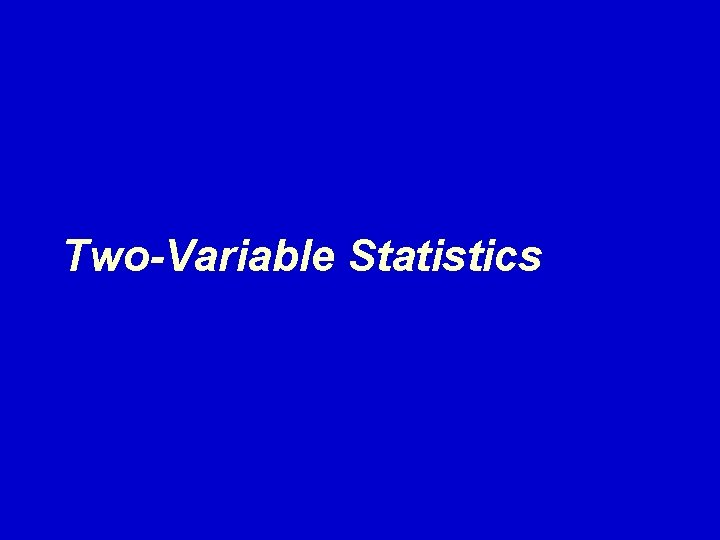Two-Variable Statistics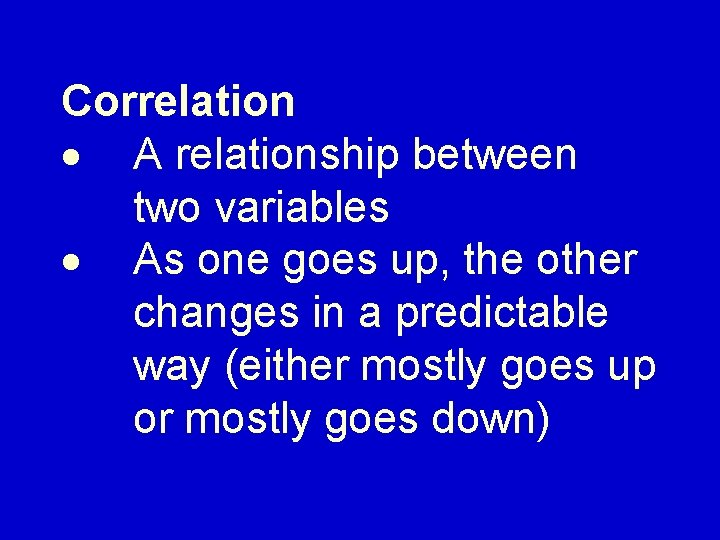Correlation · A relationship between two variables · As one goes up, the other changes in a predictable way (either mostly goes up or mostly goes down)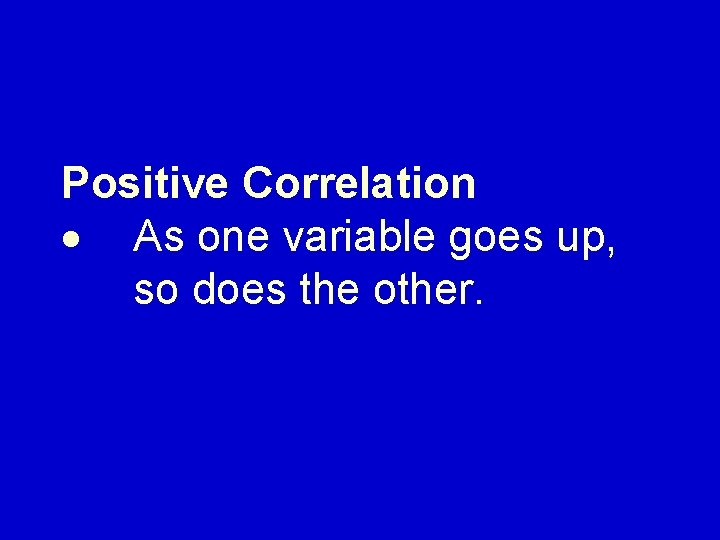Positive Correlation · As one variable goes up, so does the other.· Examples of positive correlations: cost of using a computer printer has a positive correlation with the number of pages printednumber of free throws a basketball player makes and number of times the player is fouled.time required for a reading assignment and the number of pages assigned children’s ages and the number of words in their vocabulary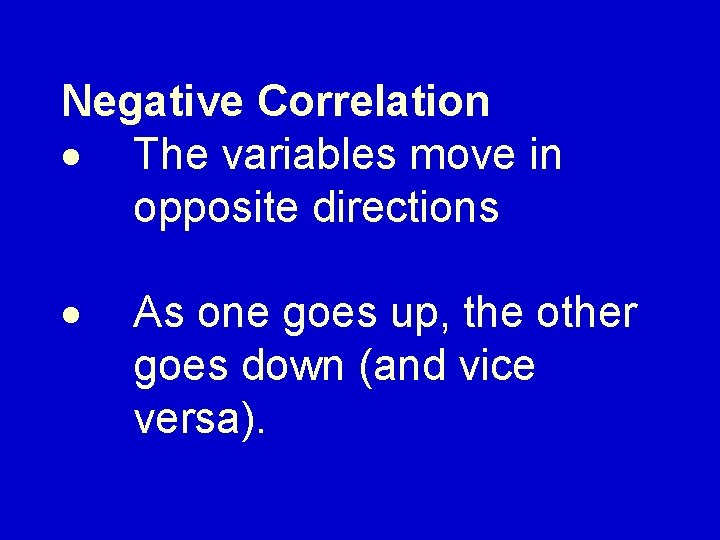Negative Correlation · The variables move in opposite directions · As one goes up, the other goes down (and vice versa).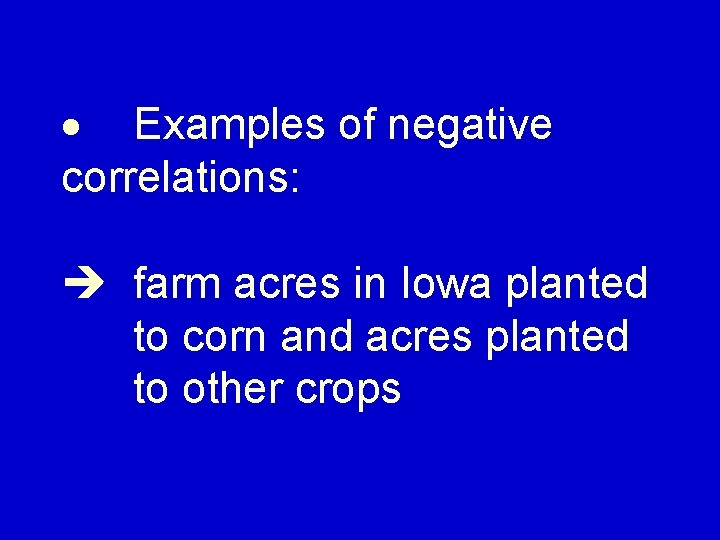· Examples of negative correlations: farm acres in Iowa planted to corn and acres planted to other cropspower of a microwave and the time it takes to boil a cup of water number of checkouts open at Wal-Mart and how long it takes to check out (at a given time of day)number of raffle tickets sold and your probability of winning the raffle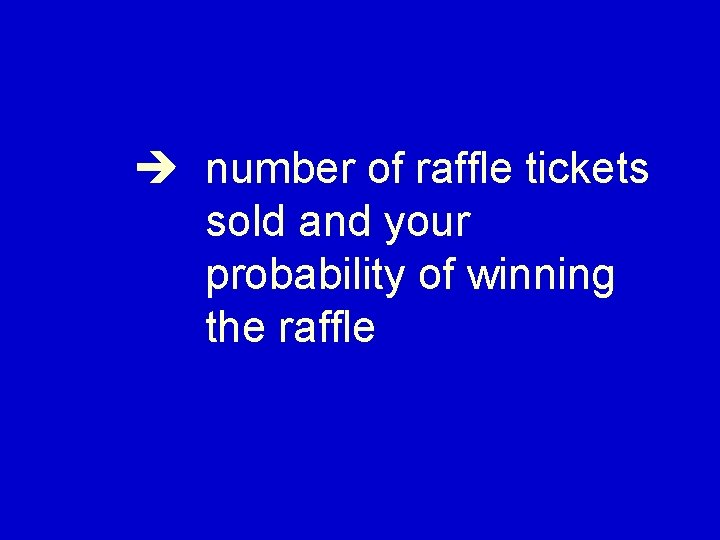number of raffle tickets sold and your probability of winning the raffle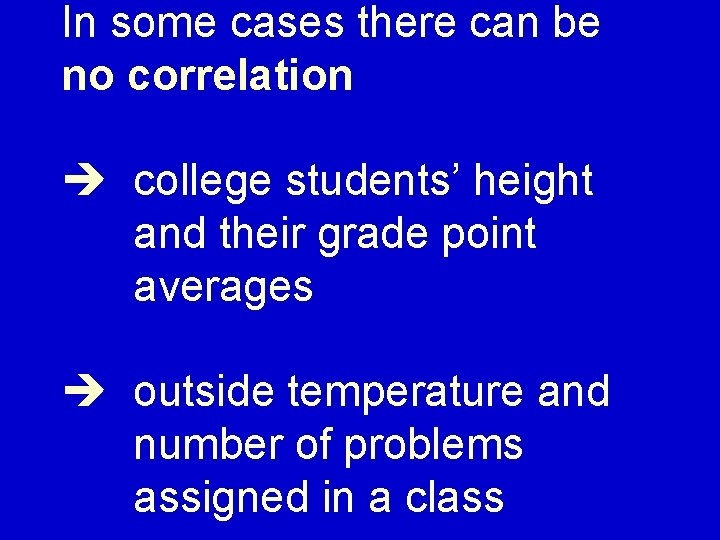In some cases there can be no correlation college students’ height and their grade point averages outside temperature and number of problems assigned in a class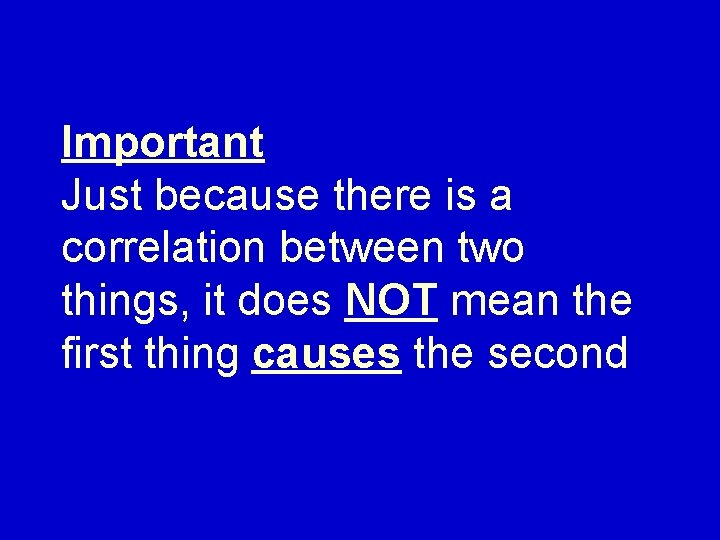Important Just because there is a correlation between two things, it does NOT mean the first thing causes the secondIt just means there’s a relationship. Something else could be affecting both of the things we are studying. This is called a lurking, confounding, or extraneous variable.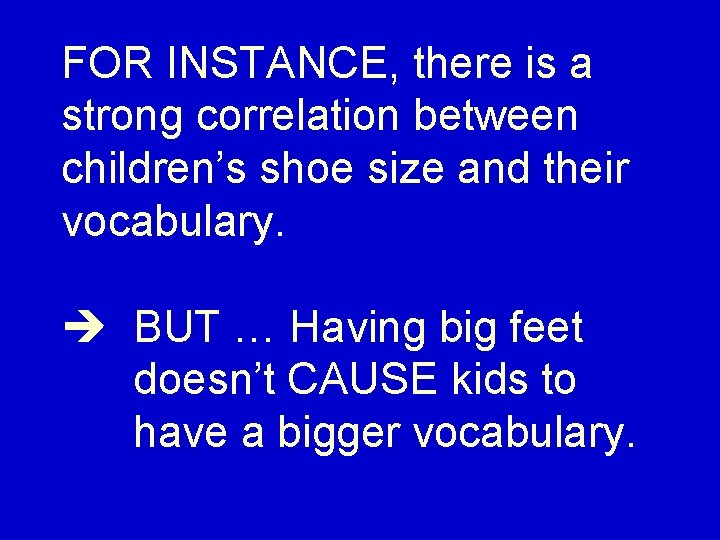FOR INSTANCE, there is a strong correlation between children’s shoe size and their vocabulary. BUT … Having big feet doesn’t CAUSE kids to have a bigger vocabulary.Correlations can be shown using a scatterplot. This is literally a graph of the points (x, y) for the values in the relationship.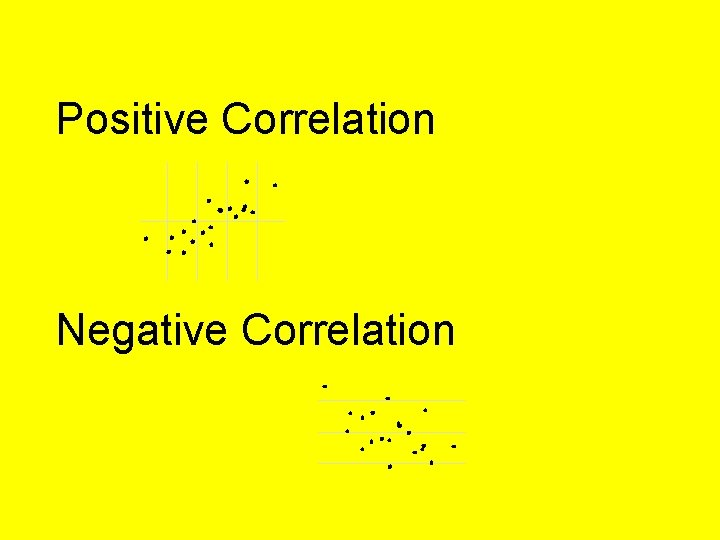Positive Correlation Negative CorrelationStrong Correlation Weak Correlation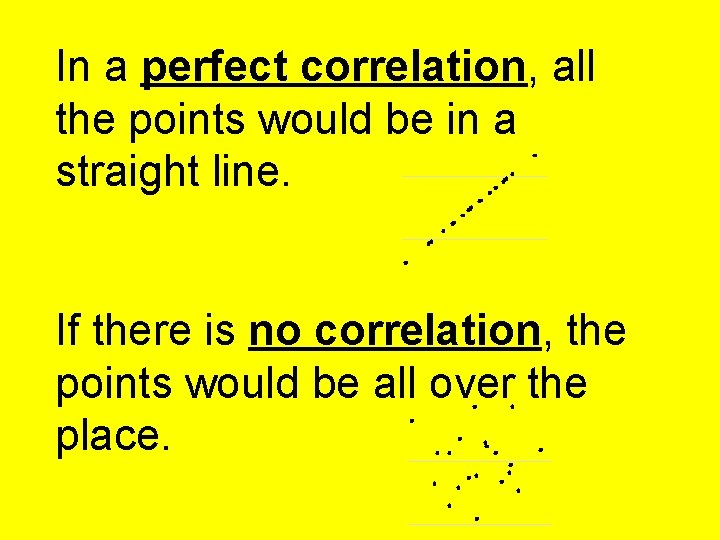In a perfect correlation, all the points would be in a straight line. If there is no correlation, the points would be all over the place.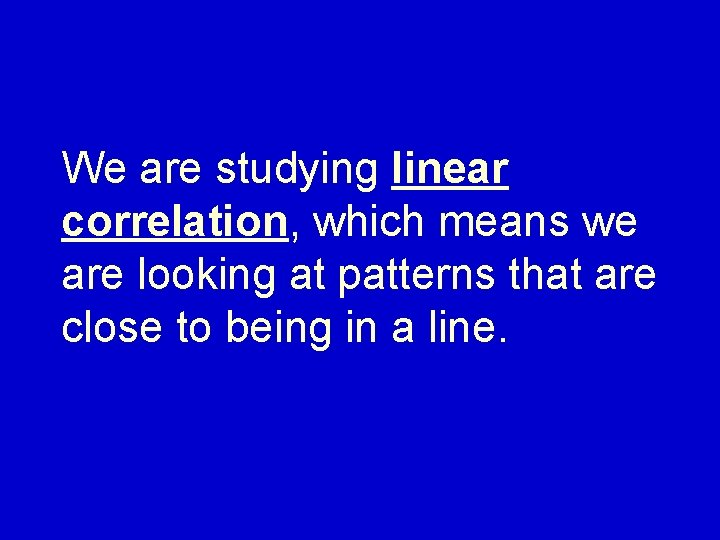We are studying linear correlation, which means we are looking at patterns that are close to being in a line.It is possible to have other patterns that don’t happen to approximate a line.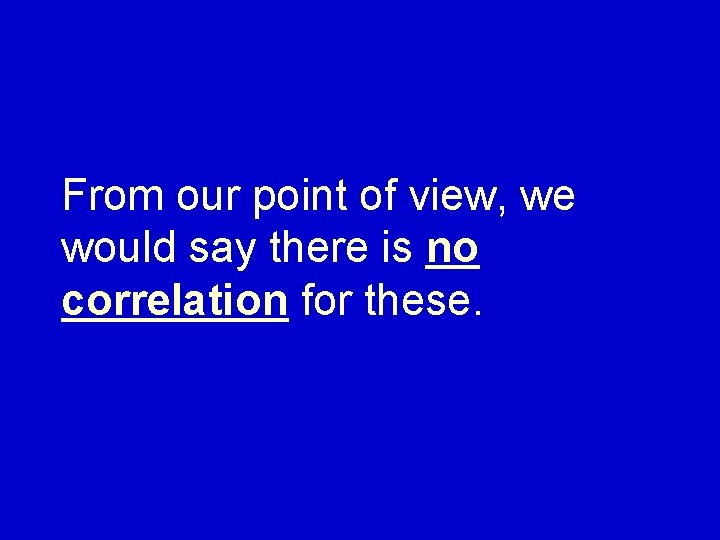From our point of view, we would say there is no correlation for these.We typically measure the strength of a correlation with a statistic called “r”.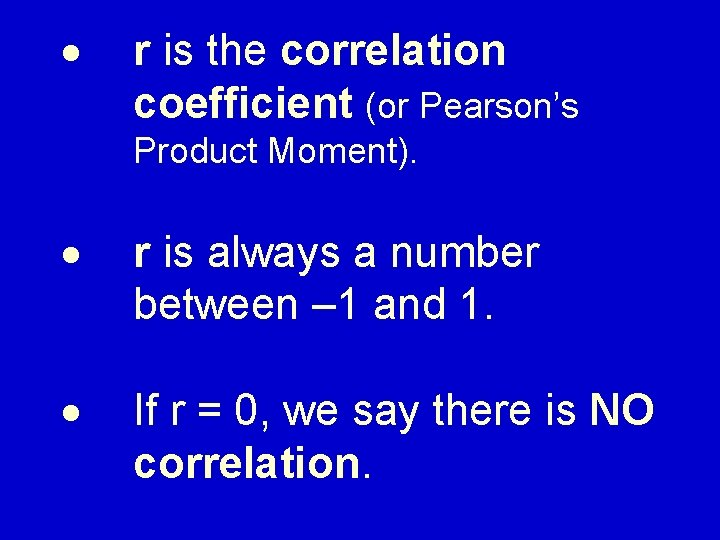· r is the correlation coefficient (or Pearson’s Product Moment). · r is always a number between – 1 and 1. · If r = 0, we say there is NO correlation.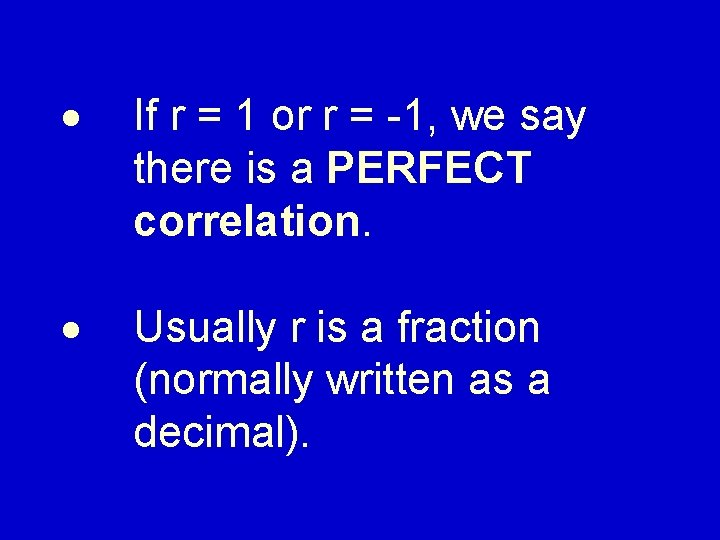· If r = 1 or r = -1, we say there is a PERFECT correlation. · Usually r is a fraction (normally written as a decimal).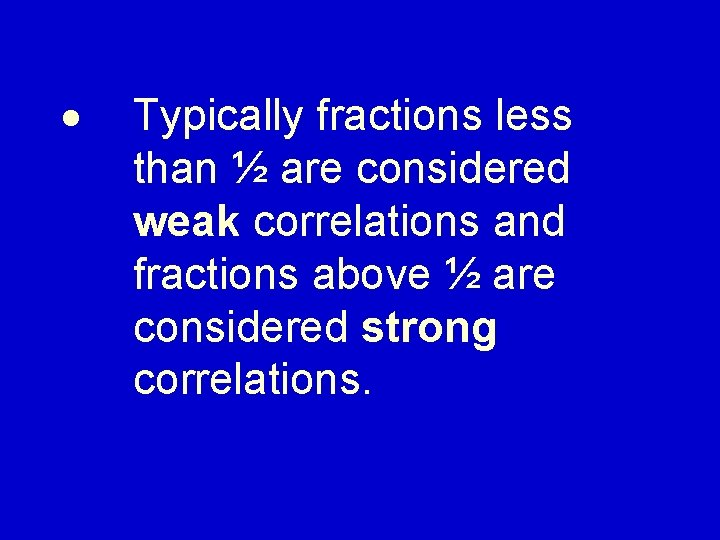· Typically fractions less than ½ are considered weak correlations and fractions above ½ are considered strong correlations.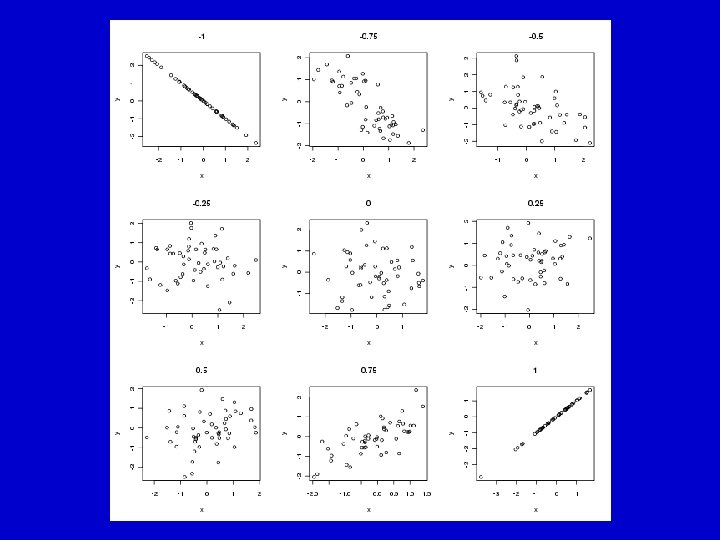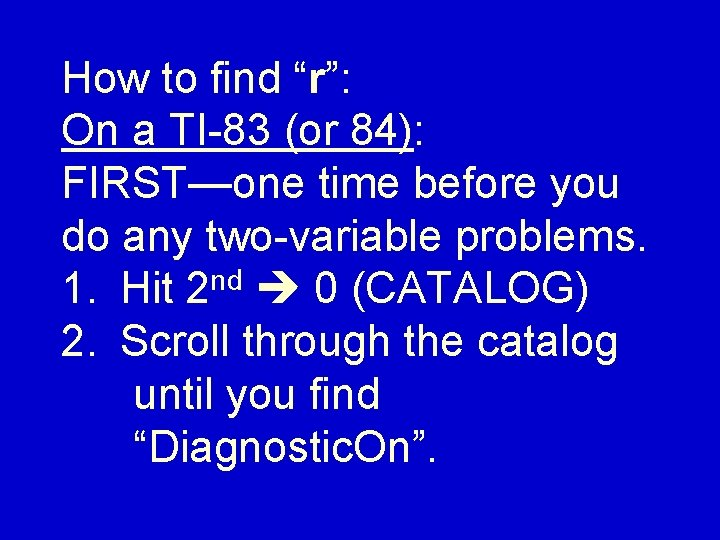How to find “r”: On a TI-83 (or 84): FIRST—one time before you do any two-variable problems. 1. Hit 2 nd 0 (CATALOG) 2. Scroll through the catalog until you find “Diagnostic. On”.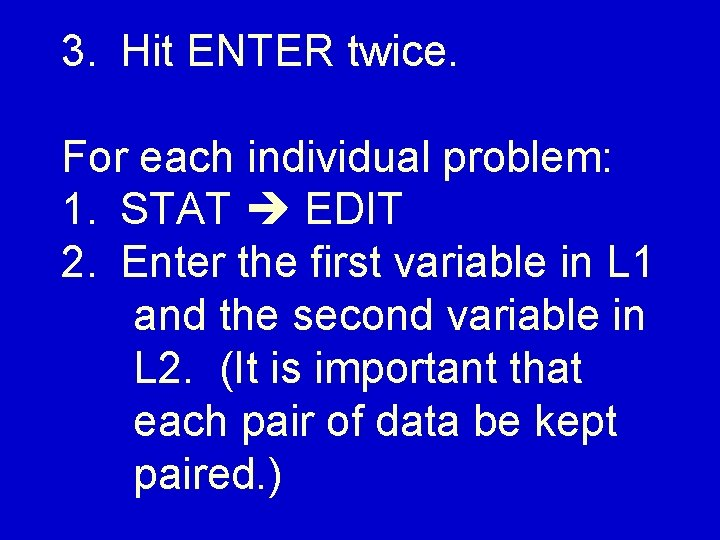3. Hit ENTER twice. For each individual problem: 1. STAT EDIT 2. Enter the first variable in L 1 and the second variable in L 2. (It is important that each pair of data be kept paired. )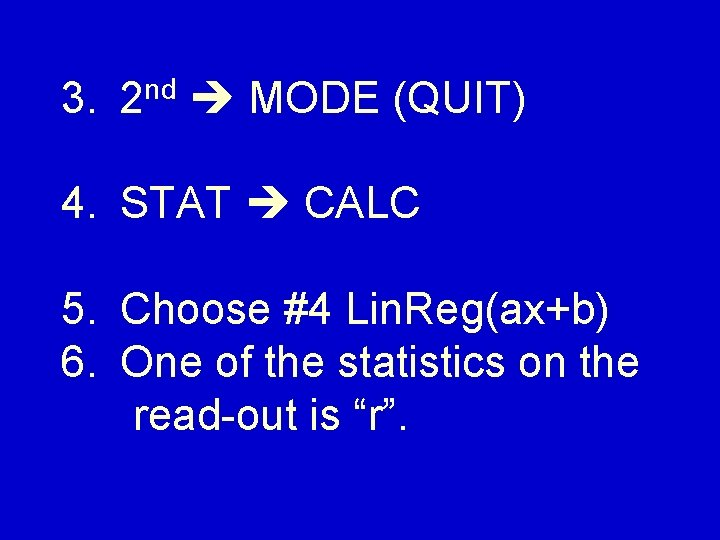3. nd 2 MODE (QUIT) 4. STAT CALC 5. Choose #4 Lin. Reg(ax+b) 6. One of the statistics on the read-out is “r”.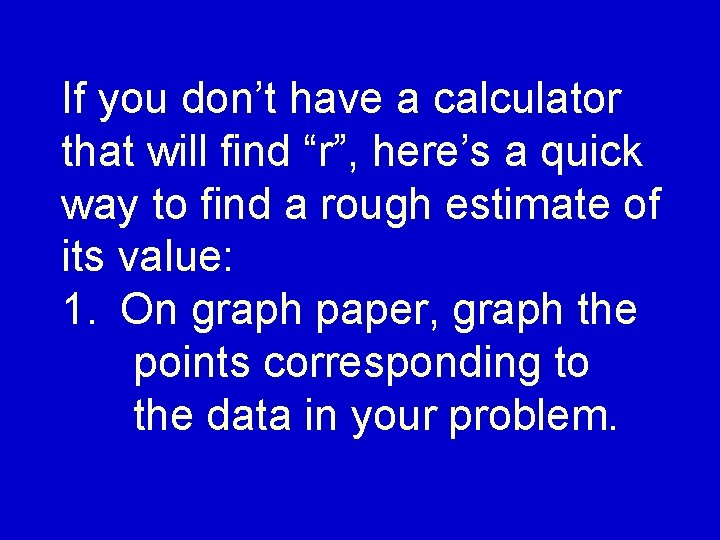If you don’t have a calculator that will find “r”, here’s a quick way to find a rough estimate of its value: 1. On graph paper, graph the points corresponding to the data in your problem.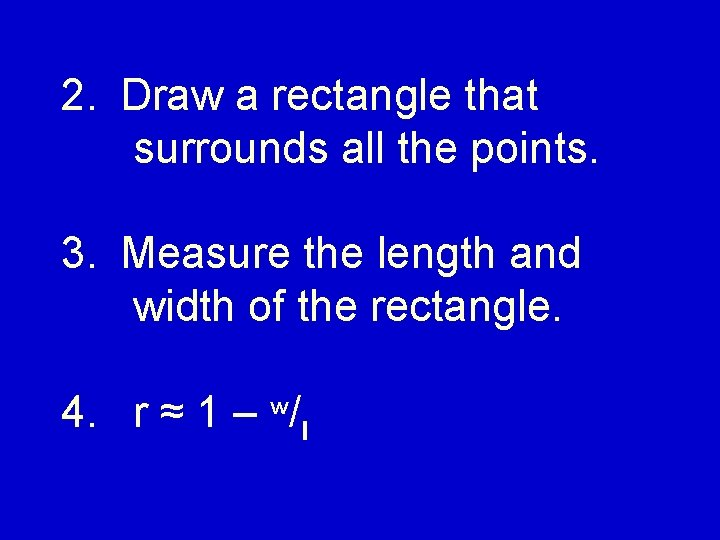2. Draw a rectangle that surrounds all the points. 3. Measure the length and width of the rectangle. 4. r ≈ 1 – w/l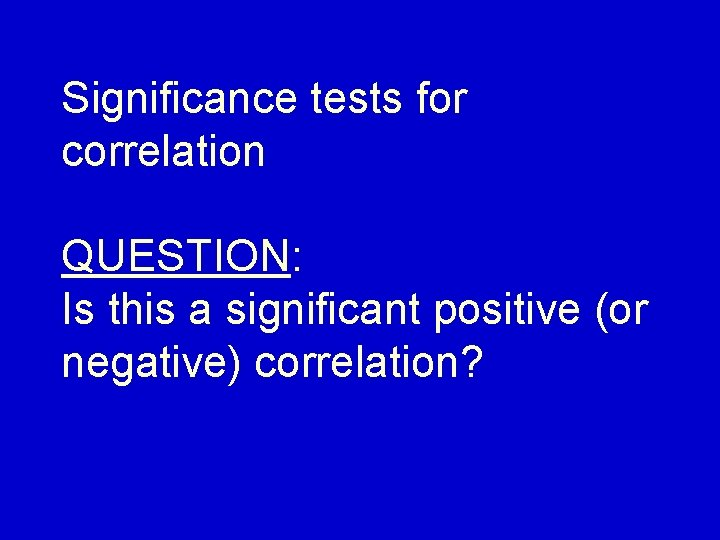Significance tests for correlation QUESTION: Is this a significant positive (or negative) correlation?HYPOTHESES: H 1 = the correlation in the whole population is significantly greater than 0. H 0 = the correlation isn’t significant.You find the critical value in the “Pearson Product Moment” table on the handout. Use the 1 -tail test for the given level of significance.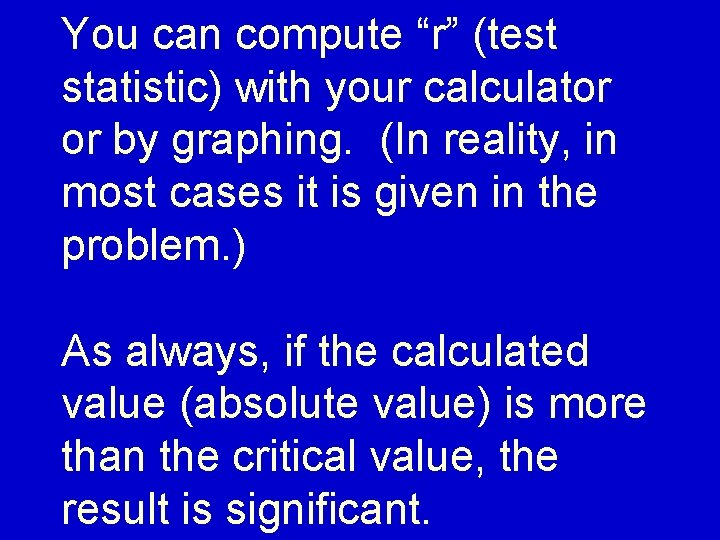You can compute “r” (test statistic) with your calculator or by graphing. (In reality, in most cases it is given in the problem. ) As always, if the calculated value (absolute value) is more than the critical value, the result is significant.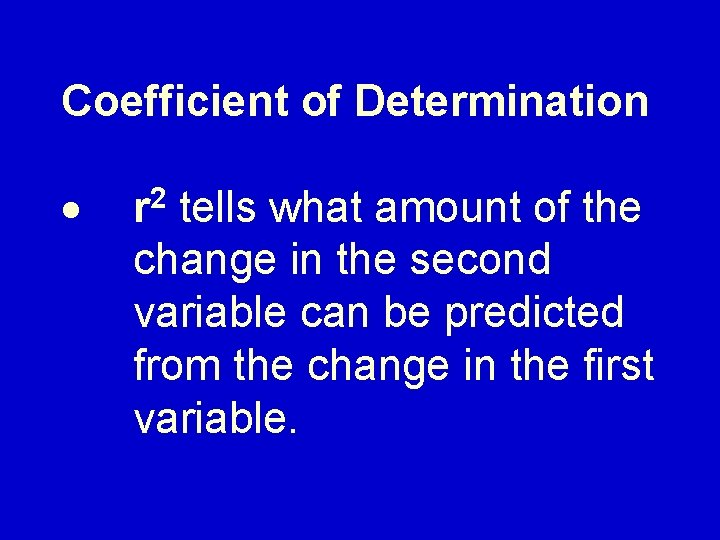Coefficient of Determination · r 2 tells what amount of the change in the second variable can be predicted from the change in the first variable.· For example, if r =. 7, then. 49 or 49% of the change in “y” can be predicted from the change in “x”.Linear Regression · using a line to make predictions with 2 -variable data · The regression line is essentially the “average” of the data.· The idea is a line that runs through the center of all the points—as close as possible to every point.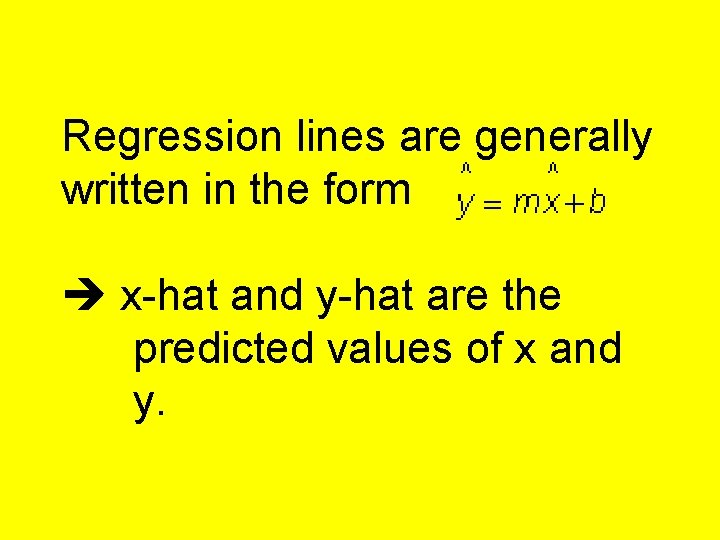Regression lines are generally written in the form x-hat and y-hat are the predicted values of x and y.On graphing calculators, the slope and y-intercept of the regression line are easily calculated. These may be given in either the form “ax + b” or “a + bx” —depending on the calculator.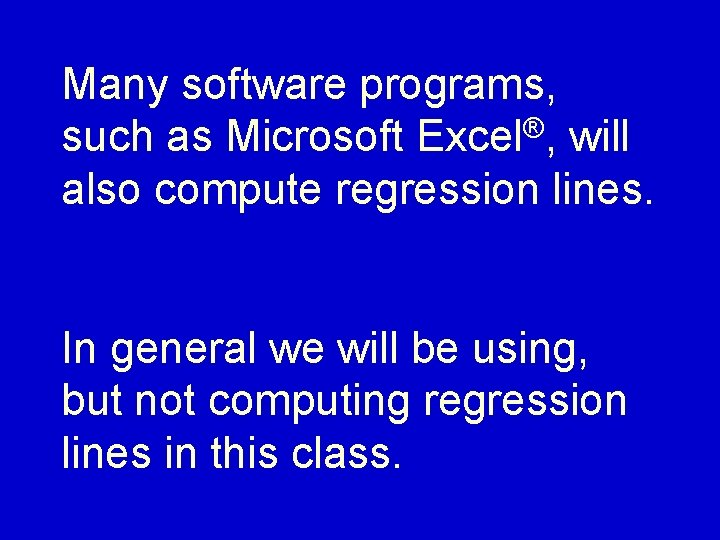Many software programs, such as Microsoft Excel®, will also compute regression lines. In general we will be using, but not computing regression lines in this class.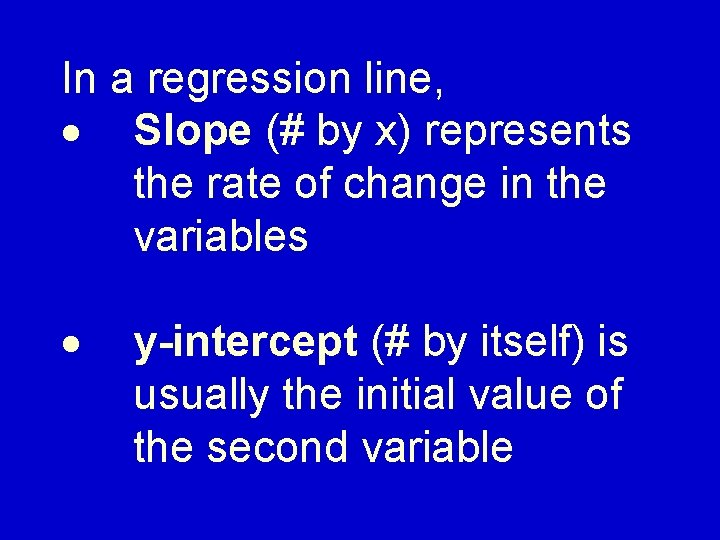In a regression line, · Slope (# by x) represents the rate of change in the variables · y-intercept (# by itself) is usually the initial value of the second variableIMPORTANT: Regression equations will give reasonable (but not exact) estimates for values near the data used in the sample.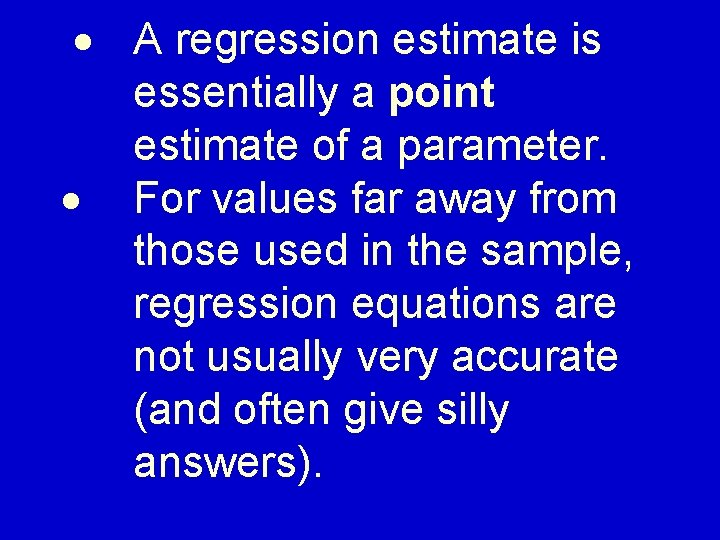· A regression estimate is essentially a point estimate of a parameter. · For values far away from those used in the sample, regression equations are not usually very accurate (and often give silly answers).Typical Problem: The New York City police noticed that as the number of officers on the street increased, the crime rate decreased.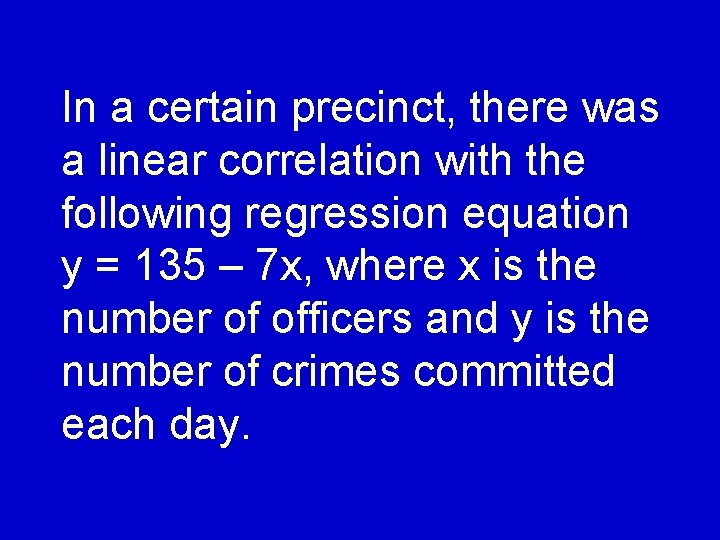In a certain precinct, there was a linear correlation with the following regression equation y = 135 – 7 x, where x is the number of officers and y is the number of crimes committed each day.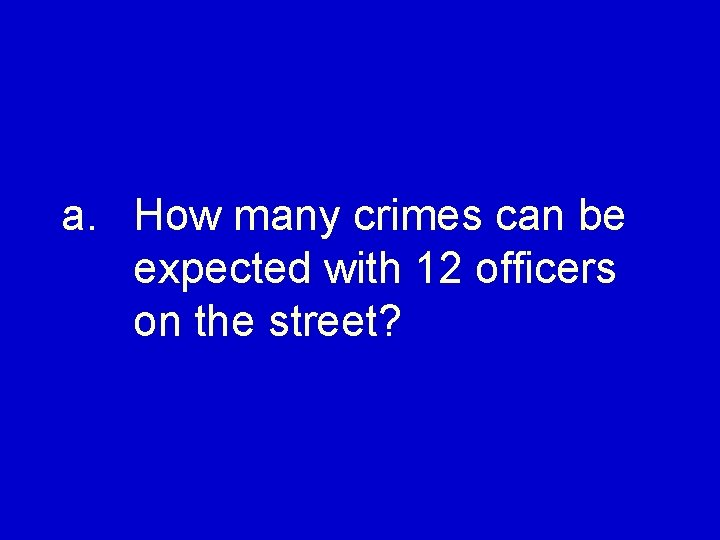a. How many crimes can be expected with 12 officers on the street?b. How many officers would be needed to reduce the number of crimes to 20?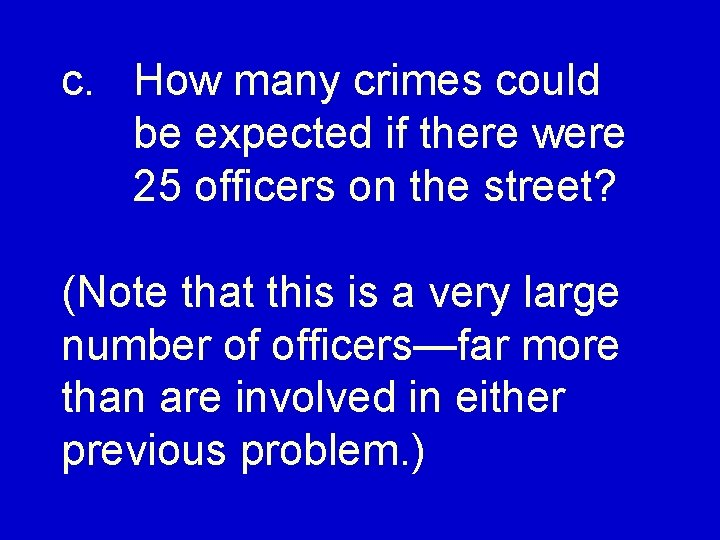c. How many crimes could be expected if there were 25 officers on the street? (Note that this is a very large number of officers—far more than are involved in either previous problem. )This is a silly answer because the data is nowhere close to the numbers used in the original sample.